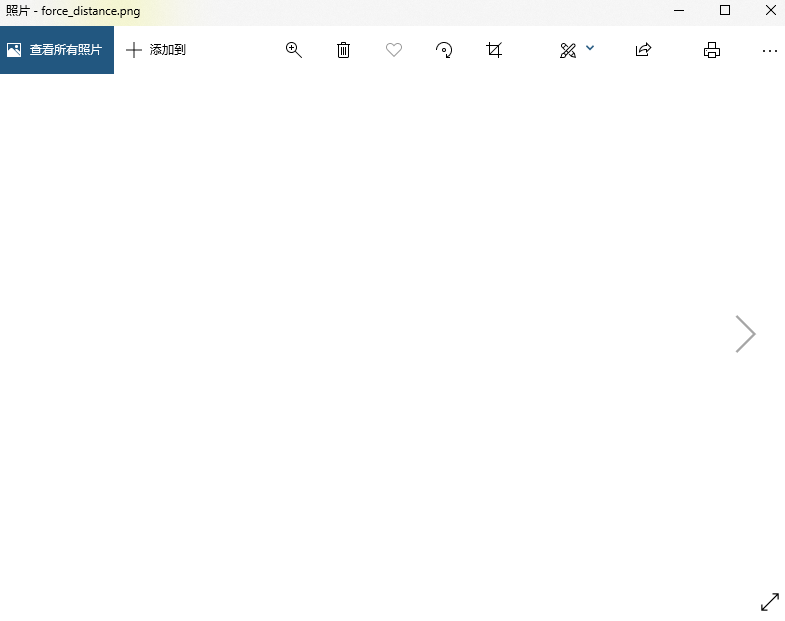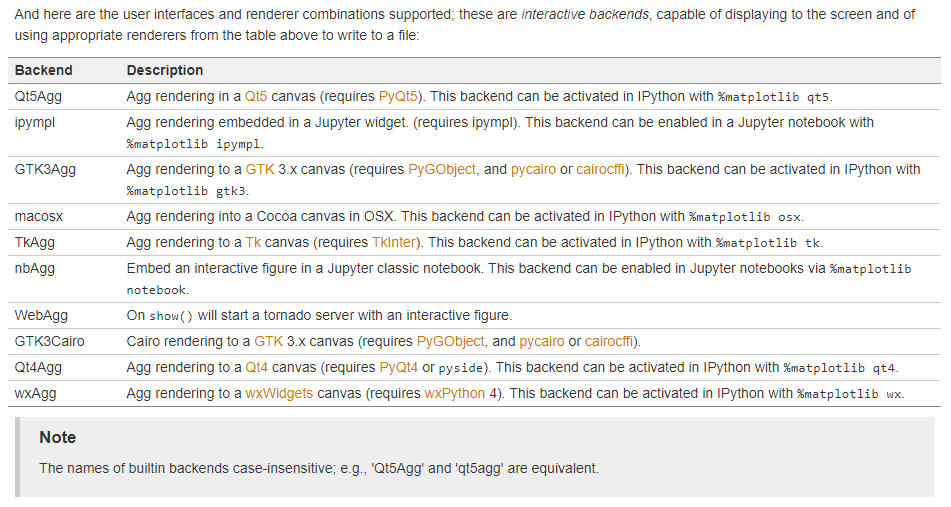﻿• 价格透明
• 信息保密
• 进度掌控
• 售后无忧# 《Python数学编程》练习-day013: matplotlib(3)

### 《Python数学编程》练习-day013: matplotlib(3)

Day1:《Python数学编程》练习-day001：斐波那契序列
Day2:《Python数学编程》练习-day002：分数操作
Day3:《Python数学编程》练习-day003：基本数学运算
Day4:《Python书序编程》练习-day004：复数
Day5:《Python书序编程》练习-day005：数值输入
Day6:《Python书序编程》练习-day006：计算整数因子
Day7:《Python书序编程》练习-day007：生成乘法表
Day8:《Python书序编程》练习-day008：测量单位转换
Day9:《Python书序编程》练习-day009：求解二次方程的根
Day11:《Python书序编程》练习-day011：matplotlib(1)
Day12:《Python书序编程》练习-day011：matplotlib(2)

### 文章目录

• 《Python数学编程》练习-day013: matplotlib(3)
• 前言
• 一、绘制牛顿万有引力定律公式图形
• 二、为什么使用matplot.use() ？
• 三、TkAgg是什么，解决哪些问题?
• 1.TkAgg:
• 四、matplotlib其他的渲染组合？
• 总结

《Python数学编程》练习-day011: matplotlib(1)

# 前言

1. 绘制公式图形；
2. 为什么使用matplot.use() ；
3. TkAgg是什么，解决哪些问题；
4. matplotlib其他的渲染组合。

# 一、绘制牛顿万有引力定律公式图形

F = G m 1 m 2 r 2 \ F=\frac{Gm_1m_2 }{r^2}

# 牛顿万有引力
# 实现质量为m1、m2两物体随距离变化，两者之间引力之间的变化——即是引力与距离之间的关系
# 实现步骤：1.绘制图形；2.定义引力计算公式：1）距离生成；2）距离对应的引力

import matplotlib.pyplot as plt
import matplotlib
# 如果您的脚本依赖于特定的后端，则可以使用功能matplotlib.use（）：
# Agg rendering to a Tk canvas (requires TkInter). This backend can be activated in IPython with %matplotlib tk.
matplotlib.use('TkAgg')

#Drew the Graph/定义一个绘图函数用于画图
def draw_graph(x, y):
plt.plot(x, y, marker = 'o')
plt.xlabel('Distance in meters(m)')
plt.ylabel('Gravitational force and distance(N)')
plt.show()
plt.savefig('C:/Users/zhangfei/Desktop/python_math/force_distance2.png')

# 定义引力公式和距离
def generate_F_r():
#Generate value for r(距离), m
r = range(20, 1001, 50)

# enpty list to store the calculated values of F, N
F = []

# Constant, G：引力常数 Nm**2kg**-2
G = 6.674*(10**-11)

# two masses, kg
m1 = 0.5
m2 = 1.5

# Calculate force and add it to the list, F：计算引力
for dist in r:
force = G*(m1*m2)/(dist**2)
F.append(force)

# call the draw_graph function(调用画图函数)
draw_graph(r, F)

if __name__ == '__main__':
generate_F_r()未使用matplotlib.use(‘TkAgg’)时，导出的图片（一片空白）：# 二、为什么使用matplot.use() ？

1. If your script depends on a specific backend you can use the function matplotlib.use():
This should be done before any figure is created, otherwise Matplotlib may fail to switch the backend and raise an ImportError.
Using use will require changes in your code if users want to use a different backend. Therefore, you should avoid explicitly calling use unless absolutely necessary.

# 三、TkAgg是什么，解决哪些问题?

### 1.TkAgg:

Agg rendering to a Tk canvas (requires TkInter). This backend can be activated in IPython with %matplotlib tk.

# 四、matplotlib其他的渲染组合？这里是原地址：Usage Guide（This tutorial covers some basic usage patterns and best-practices to help you get started with Matplotlib.）

# 总结

1. 绘制公式图形；
2. 为什么使用matplot.use() ；
3. TkAgg是什么，解决哪些问题；
4. matplotlib其他的渲染组合。
5. matplotlib使用说明。
6. 不要惧怕问题，同样不要仅仅知道解决问题的方法，还要弄懂其背后的原理。### 低价透明### 金牌服务### 信息保密